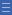# Expanding the view

Expanding the view

To avoid the limitations of the double-count perception of fractions, students need to expand their view of fractions beyond the basic part-whole model.

Use the following teaching tips to avoid or change the double-count view of fractions.

• Read fractions as single numbers rather than a part-whole description using two numbers (e.g. say 'three-quarters' instead of 'three out of four').
• Accompany part-whole models, such as area diagrams, with fractions placed on a number line.
• Strengthen students' sense of the relative size of fractions through visualising tasks. An example is the activity Fraction Wheel.
• Use 'counting by fractions' of the same denominator to reinforce the concept of fractions as numbers. An example is the activity Sequencing and Counting.
• Use sharing division tasks to explore the division meaning for fractions. An example is the activity Dividing Pancakes.
• Discuss with students what fractions actually are and the variety of ways in which they can be represented and used.

Yes

Yes

Name Class SectionYear 2: Recognise and interpret common uses of halves, quarters and eighths of shapes and collections Infobox 3Year 3: Model and represent unit fractions including 1/2, 1/4, 1/3, 1/5 and their multiples to a complete whole Infobox 3Year 4: Count by quarters halves and thirds, including with mixed numerals. Locate and represent these fractions on a number line Infobox 3Source Infobox 3